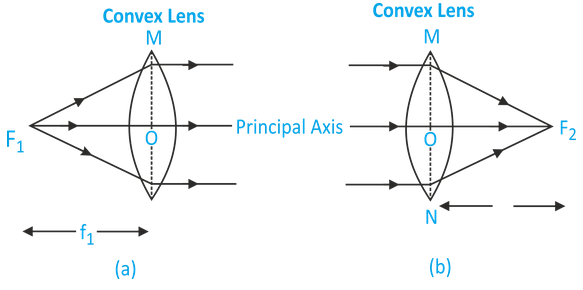# Principal Focus And Focal Length Of A Convex Lens

## PRINCIPAL FOCUS:

Principal focus of a convex lens is a point on its principal axis to which light rays parallel to the principal axis converge after refraction by the lens. It is usually represented by the letter ‘F’. However a convex lens has two spherical surfaces and hence it has two principal foci or two focal points which are usually denoted by the letters ‘F1’ and ‘F2’ and are known as the first principal focus (F1) and the second principal focus (F2). The two foci of a lens are at equal distances from the optical centre, one on either side of the lens.

Since all the light rays actually pass through the focus (or foci) of a convex lens, therefore, a convex lens has real focus (or foci).

(i) First Principal Focus (F1) : The first principal focus (F1) of a convex lens is the position of a point object on the principal axis of the lens, for which the image is formed at infinity. It is usually denoted by the letter ‘F1’ as shown in the figure (a)(ii) Second Principal Focus (F2) : The second principal focus (F2) of a convex lens is the position of an image point on the principal axis of the lens, for which the object is situated at infinity. It is usually denoted by the letter ‘F2’ as shown in the figure (b).

### FOCAL LENGTH:

The distance of the principal Focus (F1 or F2) from the optical centre ‘O’ of a lens is called its focal length. It is usually denoted by letter ‘f ‘. Since a convex lens has two principal foci, so it has two focal lengths, known as first focal length (f1) and the second focal length (f2).

• (i) First Focal length : The distance of the first principal focus of the lens from the optical centre ‘O’ of the lens is called first focal length of convex lens. It is represented by ‘f1’. i.e. OF1 = f1 .
• (ii) Second Focal length : The distance of second principal focus of the lens from the optical centre ‘O’ of the lens is called second focal length of convex lens. It is represented by ‘f2’ i.e. OF2 = f2 (figure b).

In a Convex lens f1 = f2